﻿ Vibration Analysis of Flexible Structural Components

### Vibration Analysis of Flexible Structural Components

Juraj Šarloši, Jozef Bocko

American Journal of Mechanical Engineering

## Vibration Analysis of Flexible Structural Components

Juraj Šarloši1,, Jozef Bocko1

1Department of Applied Mechanics and Mechanical Engineering, Technical University in Košice, Košice

### Abstract

This article deals with the vibration analysis of flexible structural or mechanical components. The purpose of this article is to derive the general nonlinear differential equations of motion regarding the free vibration of flexible members of uniform and/or variable cross section along their length. Unique solution methodologies are developed that simplify the solution of very complex problems.

### Cite this article:

• Juraj Šarloši, Jozef Bocko. Vibration Analysis of Flexible Structural Components. American Journal of Mechanical Engineering. Vol. 4, No. 7, 2016, pp 460-463. http://pubs.sciepub.com/ajme/4/7/42
• Šarloši, Juraj, and Jozef Bocko. "Vibration Analysis of Flexible Structural Components." American Journal of Mechanical Engineering 4.7 (2016): 460-463.
• Šarloši, J. , & Bocko, J. (2016). Vibration Analysis of Flexible Structural Components. American Journal of Mechanical Engineering, 4(7), 460-463.
• Šarloši, Juraj, and Jozef Bocko. "Vibration Analysis of Flexible Structural Components." American Journal of Mechanical Engineering 4, no. 7 (2016): 460-463.

 Import into BibTeX Import into EndNote Import into RefMan Import into RefWorks

1
Prev Next

### 1. Introduction

The main purpose in this article is to provide an introduction to the very complex problem regarding the free vibration of flexible members. Free vibrations, in general, take place from the static equilibrium position of the member. For a flexible member, the static equilibrium position is associated with large static amplitudes, and the differential equation of motion that expresses the free vibration of the member becomes nonlinear. If the vibrational amplitudes, measured from the static equilibrium position, are small, then the free frequencies of vibration are independent of the amplitude of vibration, but they do depend upon the static amplitude that defines the static equilibrium position. If, however, the frequency amplitudes are also large, then the free frequencies of vibration will be dependent on both static and vibrational amplitudes. In this article the objective is to derive the general nonlinear differential equations of motion regarding the vibration analysis of flexible members of uniform and/or variable cross section along the length of the member. The analysis in this chapter is dealing primarily with small oscillation vibration superimposed on large static displacements which define the static equilibrium position of the flexible member.

### 2. The General Nonlinear Differential Equation of Motion

We start with the Euler-Bernoulli Law. This nonlinear differential equations is as follows: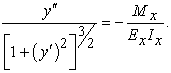(1)

By multiplying both sides of Eq. (1) by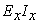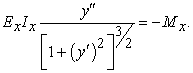(2)

Differentiating both sides of Eq. (2) with respect to x we get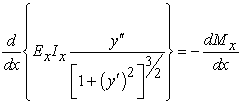(3)

or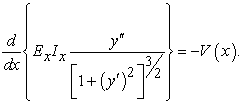(4)

The shear force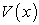at any cross section must be the same whether it is defined in the reference configuration, or deformed configuration. That is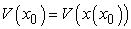.

That Eq. (4) may be written as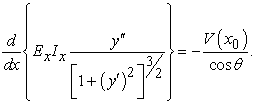(5)

By differentiating Eq. (5) with respect to x, we obtain the following expression: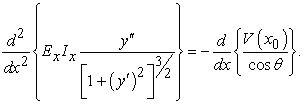(6)

Since the expression for the transverse weight is well defined in the undeformed configuration we can rewrite Eq. (6) as follows: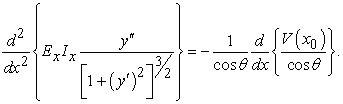(7)

For example, for distributed weight, the relation of the shear force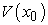to the load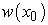is a following: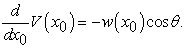(8)

Performing the indicated differentiation on the right-hand side of Eq. (7) we obtain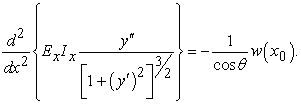(9)

Whit using the following equations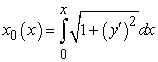(10)

and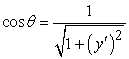(11)

we obtain for uniformly distributed loading equation where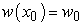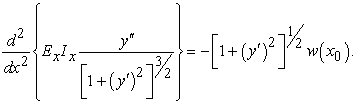(12)

For transverse free vibration, the weight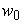is replaced by the inertia force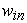.

The inertia force is express by equation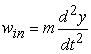(13)

where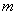is the uniform mass density, and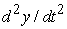is the relative acceleration.

Therefore the transverse free vibration of flexible member with a uniform distributed weight, or a distributed mass, is given by the equation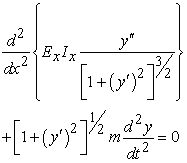(14)

In general, for arbitrarily distributed weight, the mass is replaced with an appropriate expression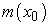. Note thatis a function of. Thus, the nonlinear differential equation of motion becomes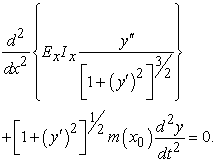(15)

Equation (15) is the one, as stated earlier, that cannot be solved be using separation of variables, and where the natural frequencies of vibration are amplitude dependent. A procedure that could be used for the solution of such problems, would be to assume the naturals of vibration and to determine the natural frequencies of vibration and the corresponding of the fundamental frequency of vibration this approach is realistic, but it becomes extremely difficult for the computation of higher frequencies of vibration.

2.1. Small Amplitude Vibration of Flexible Members

We start the derivation of the appropriate nonlinear equation of motion with small amplitude vibrations by considering the flexible cantilever beam in Figure 1a, whereis the uniform weight of the member per unit of length. However it should be realize thatmay include other weights witch are attached to the beam and participating in its vibration motion. The deformation configuration of the of the member is show in Figure 1b, where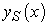is the large deformation that defines the static equilibrium position. The dynamic amplitude which represent the small vibration of the member from the static equilibrium position is denoted by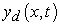. From the undeformed straight configuration of the member, the amplitude is defined by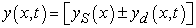(16)Download asVeiw figureFigures index
Figure 1. (a) Original undeformed flexible cantilever member. (b) Deformed configuration of uniform flexible cantilever member

In this equation, as stated earlier, is the large static deflection which defines the static equilibrium position of the member, andis the dynamic amplitude of its free vibration which is considered to be small. The slope of the dynamic amplitude curve is also small when it is compared to the large slope of the static configuration curve. This means that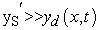. Thus, if we differentiate Eq.(16) once with respect to x, we find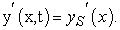(17)

By differentiate Eq. (16) twice with respect to x, we obtain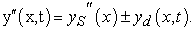(18)

Also, if we differentiate Eq.(16) twice with respect to time, we obtain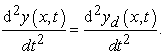(19)

The substitution of Eqs. (18) and (19), into Eq.(15) yields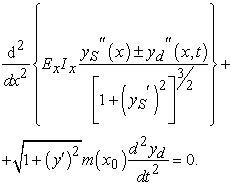(20)

From the large static equilibrium configuration, we have the expression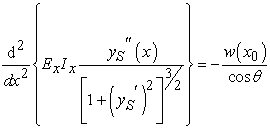(21)

and from the dynamic equilibrium configuration, we have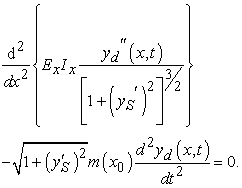(22)

Since the time function is harmonic, we can write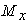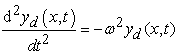(23)

where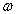is the free vibration of the flexible member in radians per second. By substituting Eq.(23) into Eq.(22), we obtain the following differential equation: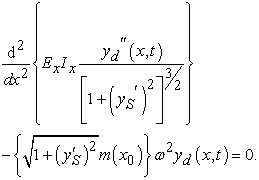(24)

From the separation of variables, Eq.(24) may also be written as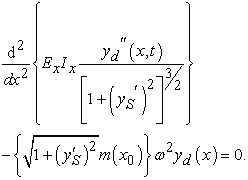(25)

We can also write Eq.(25) in terms of an equivalent variable stiffness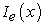and an equivalent variable mass density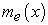as follows: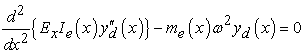(26)

where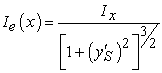(27)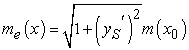(28)

and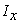is the moment of inertia of the original member at any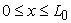. The equivalent quantitiesand, given by Eqs. (27) and (28), respectively, take into account the change in moment of inertia and mass caused by the large static deformation. Therefore the differential equation given by Eq.(26) may by thought of as representing a straight beam of length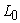, which vibrates with the same frequencies of vibration as the original member from its statics equilibrium position. The variation of its equivalent moment of inertiaand equivalent mass, along its length, are given by Eqs.(27) and (28), respectively. In summary, the transverse vibration of a flexible member subjected to large static deformationwith small amplitudes of vibration, is completely defined by the following two differential equations: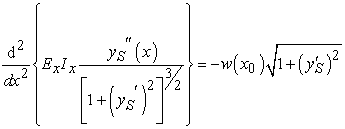(29)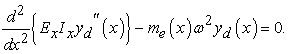(30)

Equations (29) and (30) must be solved simultaneously in order to obtain the frequencies of vibration of a flexible member. Equation (29), however, is equivalent to the Euler- Bernoulli equation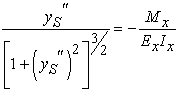(31)

whereis the banding moment of the member, andis its bending stiffness. We have to realize that bothandin Eq. (5.34) are integral equation which depend on the large static deformation of the member. We can think of Eq. (31) as representing the transverse bending vibration of a dynamically equivalent pseudo variable stiffness member of length, and having an equivalent mass density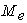and an equivalent moment of inertia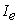. The depth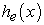of the dynamically equivalent straight member is given by the following equation: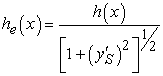(32)

where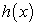represents the variation in depth of the original member. The depthof the origin member may have any arbitrary variation along its length. The solution of the differential equation of motion given by Eq. (30) may be obtained by using know linear methods of analysis for free vibration of beams. The application of the finite difference method, or utilization of the Galerkins finite element method, should yield reasonable results.

### Acknowledgements

This research was supported by grants from the Slovak Grant Agency VEGA No. 1/0731/16.

### References

  Timoshenko, History of Strength of Materials, McGraw-Hill New York, 1953.In article  I. Todhunter, K. Pearson, A History of the Theory of Elasticity and of the Strength of Materials, Vols. I and II. Dover, New York, 1960.In article  DG. Fertis, CT. Lee, Elastic and Inelastic analysis of variable thickness plates by using equivalent systems, Int. J. Eng. Struct. Mech. 1993.In article  DG. Fertis, CT. Lee, Nonlinear vibration of axially restrained elastically supported beams, In: Proc. Workshop on Dynamics, 1992.In article